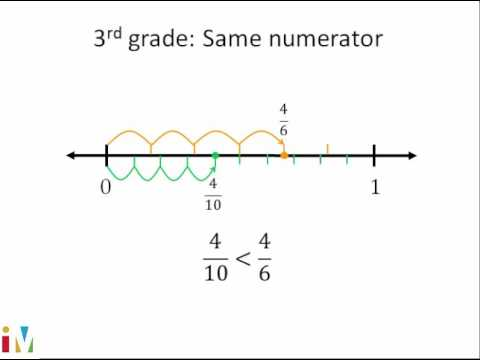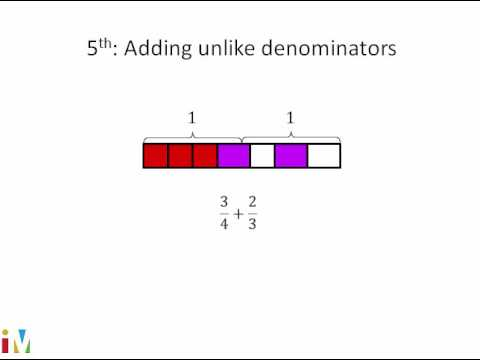Video

# More on equivalent fractions (Full video)

Khan Academy

## Supporting grades: 5

Description: Sal uses fraction models and multiplication to find equivalent fractions. Created by Sal Khan. What I want to do in this video is really digest the idea that if we have some fraction, as long as we multiply the numerator and the denominator of the fraction by the same number, then we're going to have an equivalent fraction. Let's say we multiply the denominator here by 2. I'm claiming that as long as we multiply the numerator by 2, we are going to get an equivalent fraction.

You must log inorsign upif you want to:*

*Teacher Advisor is 100% free.

### Other videos you might be interested in### Comparing Fractions

#### Illustrative Mathematics